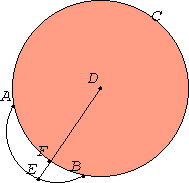# Proposition 2

If two points are taken at random on the circumference of a circle, then the straight line joining the points falls within the circle.

Let ABC be a circle, and let two points A and B be taken at random on its circumference.I say that the straight line joined from A to B falls within the circle.

III.1

For suppose it does not, but, if possible, let it fall outside, as AEB. Take the center D of the circle ABC. Join DA and DB, and draw DFE through.

Then, since DA equals DB, the angle DAE also equals the angle DBE.

I.16

And, since one side AEB of the triangle DAE is produced, the angle DEB is greater than the angle DAE.

And the angle DAE equals the angle DBE, therefore the angle DEB is greater than the angle DBE. And the side opposite the greater angle is greater, therefore DB is greater than DE. But DB equals DF, therefore DF is greater than DE, the less greater than the greater, which is impossible.

Therefore the straight line joined from A to B does not fall outside the circle.

Similarly we can prove that neither does it fall on the circumference itself, therefore it falls within.

Therefore if two points are taken at random on the circumference of a circle, then the straight line joining the points falls within the circle.

Q.E.D.

## Guide

The figure for this proposition is rather strange, but that is necessary since it refers to a hypothetical situation which is shown to be impossible. In this figure AEB is supposed to be a straight line that lies on outside the circle. There are other impossible figures in later propositions in this Book.

That Euclid even has this proposition is remarkable. Of course, it should be included, but there are equally obvious statements (but difficult to prove) left out in earlier books. For instance, that the two circles constructed in a plane on a line AB intersect is not proved although it is used in I.1. This indicates that more care has been given to the foundations for this book than for the previous books.

Euclid leaves to the reader to prove that AB cannot lie on the circumference, and that is not particularly difficult to prove.

This proposition is used in III.13.